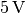# What are controlled logic gates

## Basic logic circuits¶

Basic logic circuits ("gates") form the basis for binary digital technology. They serve as conversions for the arithmetic operations NOT, AND, OR and EITHER-OR of the mathematical logic. 

The links mentioned above can be achieved in a simple manner using mechanical switches or - without mechanical components - using diodes and transistors. A distinction is then made between only two possible states:

• The HIGH state corresponds to the presence of voltage or the logical truth value "true",
• the LOW state corresponds to a lack of voltage or the logical truth value "false".

A common tension when using logic building blocks is; this voltage is also common in USB technology and when using microcontrollers (e.g. Arduinos).

### NOT circuits¶

A simple NOT circuit can be achieved with the help of a switch or button that is connected in parallel to the main circuit (in the scheme of a NOT circuit (mechanical) an LED with a series resistor). Is the switch Not closed, current flows through the main circuit - the LED lights up. If the switch is closed, the necessary operating voltage is withdrawn from the main circuit. 

A parallel connection thus reverses the normal function of the switch ("inverted"). As an alternative, a button or switch (in series) can also be installed, which is closed in the initial state and interrupts the circuit when actuated.

### AND circuits¶

A simple AND circuit can be achieved by using two switches or buttons. If these are arranged as a series circuit, current can only flow if both Switches are closed at the same time.

AND circuits in the above form are used, for example, as safety switches.

The HIGH state is only present at the output of an AND gate if the HIGH state is present at all inputs.

### OR circuits¶

A simple OR circuit can be achieved by connecting two (or more) switches or buttons in parallel to one another. A current can only flow in the main circuit if one or the other switch or both are closed at the same time.

An electronically controlled OR circuit can be implemented using two transistors. Both transistors have a common collector and emitter connection. If a positive voltage is applied to the base of one or both transistors, the main circuit is closed - the LED in the diagram of an OR circuit lights up. 

### XOR circuits¶

A mechanical XOR circuit ("exclusive" OR) can be implemented using two toggle switches with reversed pin assignment. In this way, a current flow in the main circuit is only possible when either one or the other switch is closed. If both switches are closed or open, no current can flow.

... to be continued ...

Remarks: